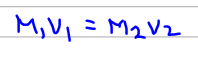# Problem: Nitric acid is usually purchased in a concentrated form that is 70.3% HNO3 by mass and has a density of 1.41 g/mL.How much concentrated solution would you take to prepare 1.15 L of 0.115 M HNO3 by mixing with water?

###### FREE Expert Solution

The problem implies that we need to calculate the volume of the concentrated solution to be able to produce a 1.15 L of 0.115 M HNO3  diluted solution.

The equation we use for dilution is shown below:Where 1 and 2 are the initial (concentrated) and final (diluted) states respectively, M= molarity and V=volume.###### Problem Details

Nitric acid is usually purchased in a concentrated form that is 70.3% HNO3 by mass and has a density of 1.41 g/mL.

How much concentrated solution would you take to prepare 1.15 L of 0.115 M HNO3 by mixing with water?